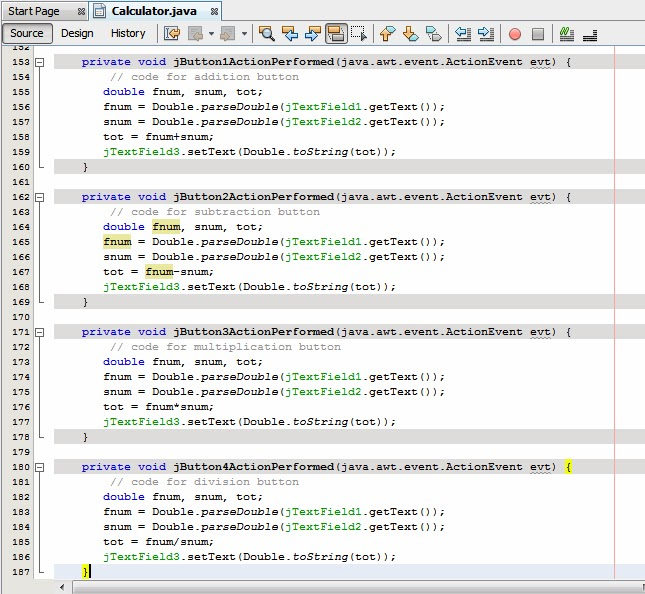# Learn How to Make a Simple Calculator in Java Language using NetBeans IDE.Calculator is used to perform various types of tasks, there are also different types of Calculator’s which you use in your day to day life, such as Scientific calculator, Loan Calculator, Simple calculator, etc. As a beginner I’ll teach you how to make a simple arithmetical calculator in Java using NetBeans IDE. The calculator you make will enable you to perform addition, Subtraction, Multiplication and Division.
Add 4 labels, 3 text fields, 4 buttons to your application by dragging them from the palette. Drag them one by one, See the below screenshot and rename them and place them in the similar way as we’ve placed.Calculator is used to perform various types of tasks, there are also different types of Calculator’s which you use in your day to day life, such as Scientific calculator, Loan Calculator, Simple calculator, etc. As a beginner I’ll teach you how to make a simple arithmetical calculator in Java using NetBeans IDE. The calculator you make will enable you to perform addition, Subtraction, Multiplication and Division.
Add 4 labels, 3 text fields, 4 buttons to your application by dragging them from the palette. Drag them one by one, See the below screenshot and rename them and place them in the similar way as we’ve placed.

• After placing them click on Addition button and write the below code

double fnum, snum, tot;

fnum = Double.parseDouble(jTextField1.getText());

snum = Double.parseDouble(jTextField2.getText());

tot = fnum+snum;

jTextField3.setText(Double.toString(tot))

• Now go to the Subtraction button and the above code but just replace the addition operator by subtracting operator, the code will look like this after you replace

double fnum, snum, tot;

fnum = Double.parseDouble(jTextField1.getText());

snum = Double.parseDouble(jTextField2.getText());

tot = fnum-snum;

jTextField3.setText(Double.toString(tot));

• Now go to the Multiplication button and replace the operator by a astrict, it refers to multiplication operator which will allow you to multiply the numbers

double fnum, snum, tot;

fnum = Double.parseDouble(jTextField1.getText());

snum = Double.parseDouble(jTextField2.getText());

tot = fnum*snum;

jTextField3.setText(Double.toString(tot));

• Now go to the Division button and replace the operator by a slash, that operator is used for dividing in computers, after replacing the code will be looking like this

double fnum, snum, tot;

fnum = Double.parseDouble(jTextField1.getText());

snum = Double.parseDouble(jTextField2.getText());

tot = fnum/snum;

jTextField3.setText(Double.toString(tot));

After adding the code in all the buttons your code will look similar to the code which I’ve shown in the below Image,

Now we’ve made the application and on running Wow you’ve made your own calculator.Now we’ve made the application and on running Wow you’ve made your own calculator.

## http:// www.cpd-india.com

Contact CPD Technologies

Training Offered by CPD Technologies